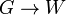# Difference between revisions of "Bruhat decomposition for general linear group of degree two over a field"

This article describes the details of the Bruhat decomposition for the general linear group of degree two over a field$K$. Let$G =GL(2,K)$ and$B$ denote the Borel subgroup of upper-triangular matrices. We have that:$G = \bigcup_{w \in W} BwB$

where$W$ is the Weyl group, which in this case is the symmetric group of degree two (which is isomorphic to cyclic group:Z2). In other words, there is a set map$G \to W$ whose fibers are the double cosets of$B$, and whose restriction to the subgroup$W$ of$G$ is the identity map. The map is well defined because every double coset of$B$ intersects$W$ at a unique point.

Note that the set map is not a homomorphism of groups.

In this case,$W$ is a set of size two, so there are only two double cosets, one being the subgroup$B$ itself (all elements in this map to the identity element of$W$ and the other being the elements of$G$ outside$B$ (these map to the non-identity element of$W$).

Another way of putting this is that there is a set map from the left coset space$G/B$ to$W$ that sends a left coset containing an element of$W$ to that element of$W$, and that is invariant under the left action of$B$ by multiplication.

## Interpretation in terms of flags

The mapping:$G/B \to W$

can be interpreted as follows: an element of$G/B$ is a complete flag of subspaces for the two-dimensional space$K^2$, and the mapping to$W$ describes its relative position with respect to the standard flag (the one stabilized by$B$). If the flag is equal to the standard flag, then the map sends it to the identity element of$W$, otherwise it is sent to the non-identity element of$W$.

## Finite field case

In the finite field case, for a finite field with$q$ elements, the fibers for the Bruhat map:$G/B \to W$

have sizes 1 and$q$ respectively. More explicitly:

Element of$W$ Expression as Bruhat word Matrix Size of fiber in$G/B$ Degree of polynomial (= Bruhat length) Size of fiber in$G$ (equals$|B|$ times size of fiber in$G/B$) Explanation$()$ empty word$\begin{pmatrix} 1 & 0 \\ 0 & 1 \\\end{pmatrix}$ 1 0$q(q-1)^2$ only the flag stabilized by$B$.$(1,2)$$s_1$$\begin{pmatrix} 0 & 1 \\ 1 & 0 \\\end{pmatrix}$$q$ 1$q^2(q-1)^2$ Number of one-dimensional subspaces other than the one used in the flag stabilized by$B$, becomes$(q + 1) - 1 = q$.
Total -- --$q + 1$ = size of$G/B$ --$q(q + 1)(q - 1)^2$ = order of$GL(2,q)$ --